Next: What do solutions look Up: The Art of Phugoid Previous: The Art of Phugoid

# The Phugoid model

The Phugoid model is a system of two nonlinear differential equations in a frame of reference relative to the plane. Let v(t) be the speed the plane is moving forward at time t, and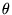(t) be the angle the nose makes with the horizontal. As is common, we will suppress the functional notation and just write v when we mean v(t), but it is important to remember that v andare functions of time.If we apply Newton's second law of motion (force = mass × acceleration) and examine the major forces acting on the plane, we see easily the force acting in the forward direction of the plane is

m= - mg sin- drag.

This matches with our intuition: Whenis negative, the nose is pointing down and the plane will accelerate due to gravity. When> 0, the plane must fight against gravity.

In the normal direction, we have centripetal force, which is often expressed as mv2/r, where r is the instantaneous radius of curvature. After noticing that that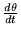= v/r, this can be expressed as v, giving

mv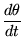= - mg cos+ lift.

Experiments show that both drag and lift are proportional to v2, and we can choose our units to absorb most of the constants. Thus, the equations simplify to the system= - sin- Rv2=which is what we will use henceforth. Note that we must always have v > 0.

It is also common to use the notation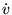for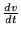and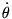for. We will use these notations interchangeably.Next: What do solutions look Up: The Art of Phugoid Previous: The Art of Phugoid

Translated from LaTeX by Scott Sutherland
2002-08-29